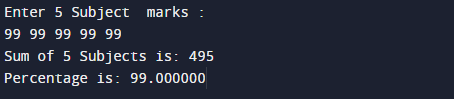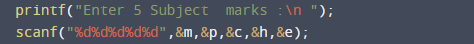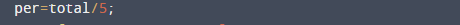C program to enter 5 subjects marks and calculate percentage.

In this tutorial you will learn about the C program to enter 5 subjects marks and calculate percentage. and its application with practical example.

In this tutorial, we will learn to create a C program that  will take five subjects marks and Calculate Percentage   using C programming.

Prerequisites

Before starting with this program  we assume that you are best aware of the following C programming topics:

• C Operators.
• Conditional statement.
• Basic input/output.

Example.

Here we will create a program in which user enter the marks of five subjects and calculate percentage. And you will learn how to find percentage of the five subjects in C language.

C Program to Calculate entered 5 subjects marks and calculate percentage.

First of all we take five number from user and add them to find total  then divide the total by 5 to get our desired percentage .Here is a program for that have a look.

OutputIn the above program, we have first declared and initialized a set variables required in the program.

• m = holds value of math.
• p = holds value of physics.
• c = holds value of chemistry
• h = holds value of Hindi.
• e= holds value of English.
• total = calculate sum of value.
• per= it will hold percentage.

In this  C program to calculate Percentage of Five Subjects .

The first printf statement will ask the user to enter the 5 subjects number.then we add all 5 given  value  in variable total ( total =m+p+c+h+e ).
Now, we calculated the percentage (per=total/5).And we will get the required output.This program allows user to enter five values of five different  subjects. And then calculate the Total and Percentage of those Five Subjects.In this tutorial we have learn about the C program to enter 5 subjects marks and calculate percentage. and its application with practical example. I hope you will like this tutorial.